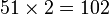# Groups of order 288

## Contents

See pages on algebraic structures of order 288| See pages on groups of a particular order

## Statistics at a glance

The number 288 has the following prime factorization:$\! 288 = 2^5 \cdot 3^2 = 32 \cdot 9$

There are only two prime factors of this number. Order has only two prime factors implies solvable (by Burnside's$p^aq^b$-theorem) and hence all groups of this order are solvable groups (specifically, finite solvable groups). Another way of putting this is that the order is a solvability-forcing number. In particular, there is no simple non-abelian group of this order.

Quantity Value List/comment
Total number of groups 1045
Number of abelian groups 14 ((number of abelian groups of order$2^5$) times ((number of abelian groups of order$3^2$) = (number of unordered integer partitions of 5) times (number of unordered integer partitions of 2) =$7 \times 2 = 14$. See classification of finite abelian groups and structure theorem for finitely generated abelian groups.
Number of nilpotent groups 102 ((number of groups of order 16) times (number of groups of order 9 =$51 \times 2 = 102$. See number of nilpotent groups equals product of number of groups of order each maximal prime power divisor, which in turn follows from equivalence of definitions of finite nilpotent group.
Number of solvable groups 1045 There are only two prime factors of this number. Order has only two prime factors implies solvable (by Burnside's$p^aq^b$-theorem) and hence all groups of this order are solvable groups (specifically, finite solvable groups). Another way of putting this is that the order is a solvability-forcing number. In particular, there is no simple non-abelian group of this order.
Number of simple groups 0 Follows from all groups of this order being solvable

## GAP implementation

The order 288 is part of GAP's SmallGroup library. Hence, any group of order 288 can be constructed using the SmallGroup function by specifying its group ID. Also, IdGroup is available, so the group ID of any group of this order can be queried.

Further, the collection of all groups of order 288 can be accessed as a list using GAP's AllSmallGroups function.

Here is GAP's summary information about how it stores groups of this order, accessed using GAP's SmallGroupsInformation function:

gap> SmallGroupsInformation(288);

There are 1045 groups of order 288.
They are sorted by their Frattini factors.
1 has Frattini factor [ 6, 1 ].
2 has Frattini factor [ 6, 2 ].
3 has Frattini factor [ 12, 3 ].
4 - 44 have Frattini factor [ 12, 4 ].
45 - 63 have Frattini factor [ 12, 5 ].
64 has Frattini factor [ 18, 3 ].
65 has Frattini factor [ 18, 4 ].
66 has Frattini factor [ 18, 5 ].
67 - 70 have Frattini factor [ 24, 12 ].
71 - 77 have Frattini factor [ 24, 13 ].
78 - 163 have Frattini factor [ 24, 14 ].
164 - 187 have Frattini factor [ 24, 15 ].
188 has Frattini factor [ 36, 9 ].
189 - 229 have Frattini factor [ 36, 10 ].
230 has Frattini factor [ 36, 11 ].
231 - 271 have Frattini factor [ 36, 12 ].
272 - 312 have Frattini factor [ 36, 13 ].
313 - 331 have Frattini factor [ 36, 14 ].
332 - 342 have Frattini factor [ 48, 48 ].
343 - 349 have Frattini factor [ 48, 49 ].
350 - 351 have Frattini factor [ 48, 50 ].
352 - 366 have Frattini factor [ 48, 51 ].
367 - 372 have Frattini factor [ 48, 52 ].
373 has Frattini factor [ 72, 39 ].
374 - 391 have Frattini factor [ 72, 40 ].
392 - 396 have Frattini factor [ 72, 41 ].
397 - 400 have Frattini factor [ 72, 42 ].
401 - 404 have Frattini factor [ 72, 43 ].
405 - 411 have Frattini factor [ 72, 44 ].
412 - 436 have Frattini factor [ 72, 45 ].
437 - 631 have Frattini factor [ 72, 46 ].
632 - 638 have Frattini factor [ 72, 47 ].
639 - 724 have Frattini factor [ 72, 48 ].
725 - 810 have Frattini factor [ 72, 49 ].
811 - 834 have Frattini factor [ 72, 50 ].
835 has Frattini factor [ 96, 226 ].
836 has Frattini factor [ 96, 227 ].
837 has Frattini factor [ 96, 228 ].
838 has Frattini factor [ 96, 229 ].
839 has Frattini factor [ 96, 230 ].
840 has Frattini factor [ 96, 231 ].
841 - 843 have Frattini factor [ 144, 182 ].
844 - 858 have Frattini factor [ 144, 183 ].
859 - 860 have Frattini factor [ 144, 184 ].
861 - 867 have Frattini factor [ 144, 185 ].
868 - 890 have Frattini factor [ 144, 186 ].
891 - 895 have Frattini factor [ 144, 187 ].
896 - 906 have Frattini factor [ 144, 188 ].
907 - 917 have Frattini factor [ 144, 189 ].
918 - 928 have Frattini factor [ 144, 190 ].
929 - 941 have Frattini factor [ 144, 191 ].
942 - 978 have Frattini factor [ 144, 192 ].
979 - 985 have Frattini factor [ 144, 193 ].
986 - 987 have Frattini factor [ 144, 194 ].
988 - 1002 have Frattini factor [ 144, 195 ].
1003 - 1017 have Frattini factor [ 144, 196 ].
1018 - 1023 have Frattini factor [ 144, 197 ].
1024 - 1045 have trivial Frattini subgroup.

For the selection functions the values of the following attributes
are precomputed and stored:
IsAbelian, IsNilpotentGroup, IsSupersolvableGroup, IsSolvableGroup,
LGLength, FrattinifactorSize and FrattinifactorId.

This size belongs to layer 2 of the SmallGroups library.
IdSmallGroup is available for this size.